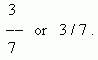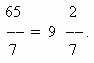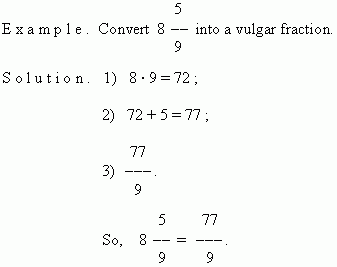# Vulgar (simple) fractions

Vulgar fraction (denominator, numerator).
Proper fraction. Improper fraction.
Mixed number (integer and fractional parts).
Converting of a mixed number into a vulgar
improper fraction and back. Reciprocal fractions.

A part of a unit or some equal parts of a unit is called a vulgar (simple) fraction . A number of equal parts into which a unit has been divided, is called a denominator ; a number of these taken parts, is called a numerator . A fraction record:Here 3 – a numerator, 7 – a denominator.
If a numerator is less than a denominator, then the fraction is less than 1 and called a proper fraction. If a numerator is equal to a denominator, the fraction is equal to 1. If a numerator is greater than a denominator, the fraction is greater than 1. In both last cases the fraction is called an improper fraction. If a numerator is divisible by a denominator, then this fraction is equal to a quotient:  63 / 7 = 9.  If a division is executed with a remainder, then this improper fraction can be presented as a mixed number:Here  9 – an incomplete quotient  ( an integer part of the mixed number ), 2 – a remainder  ( a numerator of the fractional part ),  7 – a denominator .
It is often necessary to solve a reverse problem  –  to convert a mixed number into a  fraction. For this purpose, multiply an integer part of a mixed number by a denominator and add a numerator of a fractional part. It will be a numerator of a vulgar fraction, and its denominator is saved the same.Reciprocal fractions are two fractions whose product is 1. For example,  3 / 7 and 7 / 3 ;  15 / 1 and 1 / 15 and so on.